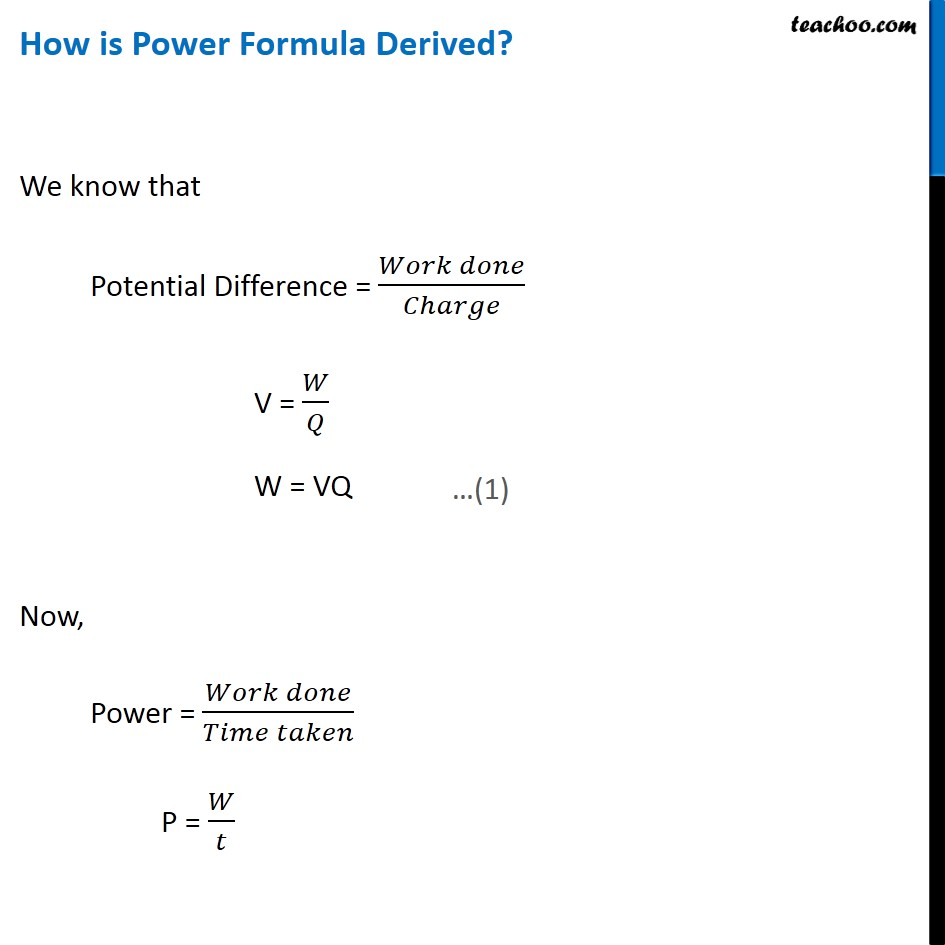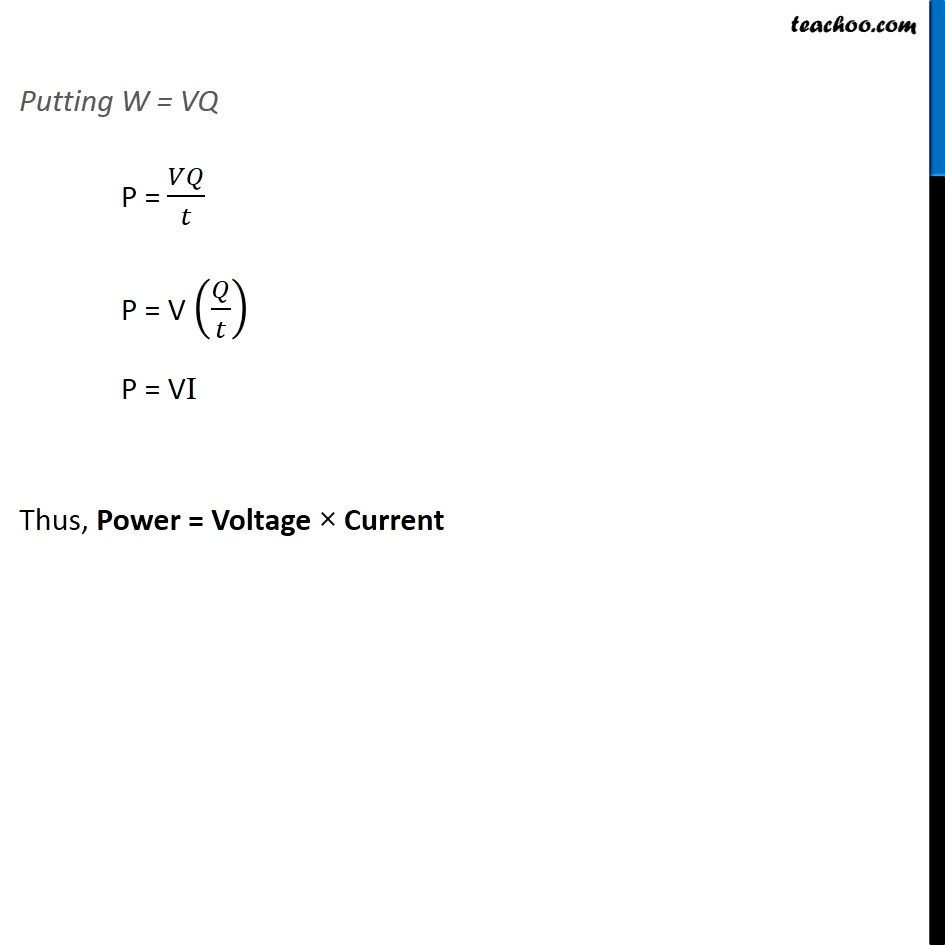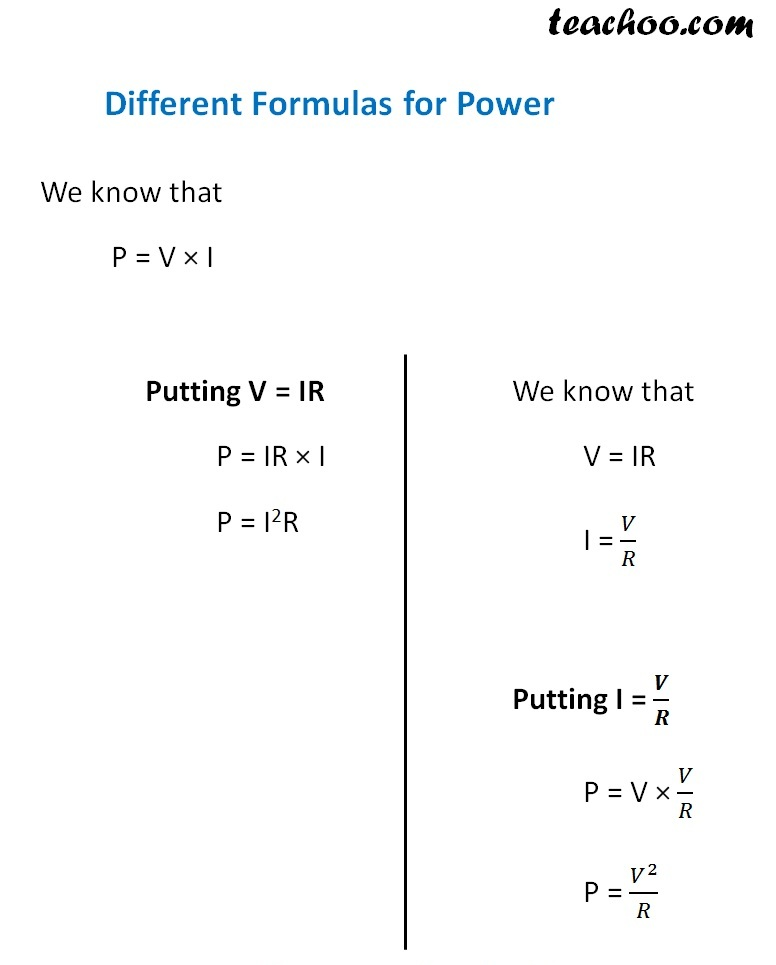Concepts

Class 10
Chapter 12 Class 10 - Electricity

## What is Power

We have studied in last class that

Rate of Doing Work is called Power

Power = Work Done/Time Taken

It is measured in Joule per second

Example

Suppose 20 Joules of work is done in 4 seconds

Power =Work Done/Time Taken

=20 Joules/4 Seconds

= 5 Joules/Second

## What is Electric Power

Rate at which electric energy is consumed in a electrical circuit

Power = VI

## How is Power Formula Derived## What is SI unit of Power

It is Watt

1 Watt = 1 Volt × 1 Ampere

1 watt is power generated by Electrical Device when

When an electrical appliance is operated at Potential Difference Of 1 Volt and a current of 1A flows through it.

## Different formulas for Calculating Power## What is Commercial Unit of Electric Power

It is measured in Kilowatt Hour (KWh)

Kilowatt hour

1 Kilowatt hour = 1000 Watt × 3600 Second

1 Kilowatt hour = 3600000 Watt Second

1 Kilowatt hour = 3.6 × 10 6 watt seconds

1 Kilowatt hour = 3.6 × 10 6 Joule

## Questions

NCERT Question 2 - Which of the following terms does not represent electrical power in a circuit?
(a) I 2 R (b) IR 2 (c) VI (d) V 2 /R

NCERT Question 3 - An electric bulb is rated 220 V and 100 W. When it is operated on 110 V, the power consumed will be –
(a) 100 W (b) 75 W (c) 50 W (d) 25 W

NCERT Question 12 - Several electric bulbs designed to be used on a 220 V electric supply line, are rated 10 W. How many lamps can be connected in parallel with each other across the two wires of 220 V line if the maximum allowable current is 5 A?

NCERT Question 14 - Compare the power used in the 2 Ω resistor in each of the following circuits:
(i) a 6 V battery in series with 1 Ω and 2 Ω resistors, and (ii) a 4 V battery in parallel  with 12 Ω and 2 Ω resistors.

NCERT Question 15 - Two lamps, one rated 100 W at 220 V, and the other 60 W at 220 V, are connected in parallel to electric mains supply. What current is drawn from the line if the supply voltage is 220 V?

NCERT Question 16 - Which uses more energy, a 250 W TV set in 1 hr, or a 1200 W toaster in 10 minutes?

NCERT Question 17 - An electric heater of resistance 8 Ω draws 15 A from the service mains 2 hours. Calculate the rate at which heat is developed in the heater.

Q1 Page 220 - What determines the rate at which energy is delivered by a current?

Q2 Page 220 - An electric motor takes 5 A from a 220 V line. Determine the power of the motor and the energy consumed in 2 h

Example 12.12 - An electric bulb is connected to a 220 V generator. The current is 0.50 A. What is the power of the bulb?

Example 12.13 - An electric refrigerator rated 400 W operates 8 hour/day. What is the cost of the energy to operate it for 30 days at Rs 3.00 per kW h?

Example 12.10 - An electric iron consumes energy at a rate of 840 W when heating is at the maximum rate and 360 W when the heating is at minimum. The voltage is 220 V. What are the current and the resistance in each case?

Question 13 - Find out the following in the electric circuit given in Figure 12.9
(a) Effective resistance of two 8 W resistors in the combination
(b) Current flowing through 4 W resistor
(c) Potential difference across 4 W resistance
(d) Power dissipated in 4 W resistor
(e) Difference in ammeter readings, if any.

Learn in your speed, with individual attention - Teachoo Maths 1-on-1 Class

### Transcript

How is Power Formula Derived? We know that Potential Difference = (𝑊𝑜𝑟𝑘 𝑑𝑜𝑛𝑒)/𝐶ℎ𝑎𝑟𝑔𝑒 V = 𝑊/𝑄 W = VQ Now, Power = (𝑊𝑜𝑟𝑘 𝑑𝑜𝑛𝑒)/(𝑇𝑖𝑚𝑒 𝑡𝑎𝑘𝑒𝑛) P = 𝑊/𝑡 …(1) Putting W = VQ P = 𝑉𝑄/𝑡 P = V (𝑄/𝑡) P = VI Thus, Power = Voltage × Current Different Formulas for Power We know that P = V × I Putting V = IR P = IR × I P = I2R We know that V = IR I = 𝑉/𝑅 Putting I = 𝑽/𝑹 P = V × 𝑉/𝑅 P = 𝑉^2/𝑅